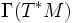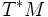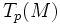Difference between revisions of "Differential 1-form"

This article defines a basic construct that makes sense on any differential manifold
View a complete list of basic constructs on differential manifolds

Definition

Symbol-free definition

A differential 1-form on a differential manifold is defined in the following ways:

• It is a section of its cotangent bundle
• It associates in a smooth manner, a linear functional on the tangent space at every point

We can also talk of a differential 1-form restricted to an open subset, which is a section of the restriction of the cotangent bundle to the open subset. This gives rise to the notion of the sheaf of differential 1-forms

Definition with symbols

Let$M$ be a differential manifold. A differential 1-form on$M$ is defined in the following equivalent ways:

• It is an element of$\Gamma(T^*M)$. Here$T^*M$ denotes the cotangent bundle of$M$
• It associates, to every point$p \in M$, a linear functional on$T_p(M)$, in a smooth manner
• it is a smooth map from$TM$ to$\R$, such that the restriction to any fiber$T_p(M)$, is a linear map.

Related notions

There is a related notion of a vector space-valued differential 1-form. This is the analog of differential 1-form, taking values in a vector space, rather than in real numbers.